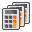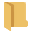RSWebinar: Designing Cost-Effective Ground Improvement Solutions with Settle3 | June 7th, 2023 Register Now

# Batch Compute

Batch Compute can quickly generate results for several UnWedge model files.

To access the Batch Compute feature of UnWedge:

1. Select File > Batch Computefrom the menu.
2. The Batch Compute dialog will appear allowing you to:
• Add files to the File Queue for computing
• See the progress of Completed Files
• See if any issues occurred during reading, computing, or writing under Warnings

## Input File

Batch Compute several Deterministic, and/or Probabilistic Analysis models using saved UnWedge (*.weg, *.weg5) model files.

To add UnWedge model files to the File Queue:

• Select the Openbutton to choose individual model files.

## Output File

When Batch Compute is run, a directory by the same name as the input model file will be created in the same directory as the input model file. This directory contains the computed results for the input model file.

The results of each model file are output to Excel (*.xlsx) format. One "Results" file is generated per model computed.

In a Probabilistic Analysis where more than one tunnel can be considered, the output file is formatted to write one tunnel analysis per row.

The output file contains 12 sheets:

• General
• Field Stress
• One sheet per wedge (8 possible Perimeter Wedges, 2 possible End Wedges)

### GENERAL DATA

The General sheet contains the following global data:

• Tunnel ID
• Tunnel Trend
• Tunnel Plunge
• Tunnel Length
• Design FS
• Rock Unit Weight
• Water Unit Weight
• Seismic Coeff.
• Seismic Coeff. X/North
• Seismic Coeff. Y/East
• Seismic Coeff. Z/Down
• Seismic Trend
• Seismic Plunge

### FIELD STRESS DATA

The Field Stress sheet contains the following global data:

• Tunnel ID
• Sigma 1
• Sigma 1 Trend
• Sigma 1 Plunge
• Sigma 2
• Sigma 3
• Sigma 3 Trend
• Sigma 3 Trend
• Ground Surface Elev.
• Major Hor. Stress Tend
• Major Hor. Stress Ratio
• Minor Hor. Stress Ratio
• Young's Modulus
• Poisson Ratio
• Num Elements
• Num Joint Sample Pts.

### WEDGE DATA

Each Wedge sheet contains the following wedge-specific data:

• Tunnel ID
• Wedge Name and Number
• Factor of Safety
• Wedge Volume
• Wedge Weight
• Wedge z-Length
• Excavation Face Area
• Support Pressure
• Resisting Force
• Driving Force
• Shear Force
• Apex Height
• Apex Angle
• Scaling Parameter
• Scaled By
• Failure Mode
• Sliding Joint Orientations
• Sliding Direction (East, North, Up)
• Sliding Direction (Trend, Plunge)
• Joint Codes, for all three joints
• Joint Dip, for all three joints
• Joint Dip Direction, for all three joints
• Friction Angle, for all three joints
• Cohesion, for all three joints
• Tensile Strength, for all three joints
• JRC, for all three joints
• JCS, for all three joints
• PhiR, for all three joints
• Power Curve A, for all three joints
• Power Curve B, for all three joints
• Power Curve C, for all three joints
• Power Curve D, for all three joints
• Waviness, for all three joints
• Water Pressure, for all three joints
• Water Elevation, for all three joints
• Joint Area, for all three joints
• Joint Persistence, for all three joints
• Joint Trace Length, for all three joints
• Joint Normal Force, for all three joints
• Joint Water Force, for all three joints
• Joint Shear Strength, for all three joints

#### Notes:

• If no valid wedge is formed, Factor of Safety = N/A and any computed values will also be N/A.
• The applicability of some computed values depends on the geometry, strength, force, and water options selected.